## Accelerate the Discrete Fourier Transform with NXP SPT

cancel
Showing results for
Did you mean:

## Accelerate the Discrete Fourier Transform with NXP SPT

2,021 ViewsNXP Employee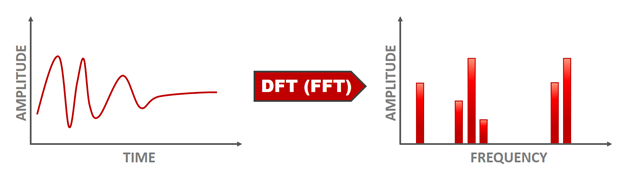Theoretical background

A Discrete Fourier Transform (DFT) converts a signal from time domain (left) into frequency domain (right). Every signal is made of one or multiple frequencies. If you consider a sound wave, then any sample of that sound wave has a set of frequencies with corresponding amplitudes that describe the overall sound wave.

Fourier demonstrated that you can take a set of sinusoids of different amplitudes and frequencies and by adding them together you can build up any signal - including a square one if needed.  A plot of frequency versus amplitude on an x-y graph of these sinusoids represents a frequency spectrum, or frequency domain plot.

# INTRODUCTION

In this article we are going to discuss about how to compute the DFT of real signals using the FFT (Fast Fourier Transform) algorithm. The FFT algorithm is implemented using the instructions RDX2 and RDX4.

To explain why we need to compute the DFT in RADAR, let us take the simplest scenario. Suppose that we have only one object in front of the RADAR, then the demodulated signal from a chirp is a sinusoid. The frequency of the sinusoid is proportional to the range between the RADAR and the object. To find the frequency of the sinusoid we compute its DFT. For positive frequencies the DFT will have only one peak. The peak is at the frequency of the sinusoid.

# SPT INPUT DATA

Data received by the SPT is made of demodulated signal and can be organized as a 3-dimensional array in the following way: there is a page for each antenna, and each page contains a matrix where each column contains the samples of a chirp.

`In MATLAB we refer to the third dimension as pages. For example v(:,:,1) is the first page of v.         `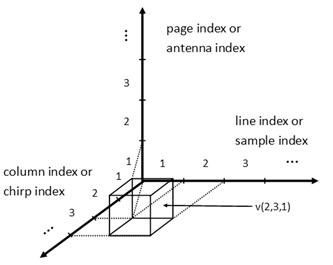Fig. 1: SPT input data representation

% Number of bits used to retain the operands

NBITS = 16;

% Number of samples per chirp

M = 512;

% Number of chirps

N = 256;

% Number of antennas

P = 4;

% We take a input a 3D sine wave. This is the case when there is only one

% object in front of the radar.

% The frequency in the first dimension or the frequency of each chirp

fs = 50.5 / M;

% The frequency in the second dimension or the Doppler frequency

fc = -99.5 / N;

% The frequency in the third dimension

fa = 1.5 / P;

ts = (0:M-1)';

tc = 0:N-1;

ta(1, 1, : )= 0:P-1;

ts = repmat(ts, [1, N, P]);

tc = repmat(tc, [M, 1, P]);

ta = repmat(ta, [M, N, 1]);

% Input data

input_data = sin(2*pi*(fs*ts + fc*tc + fa*ta));

% Input data as 16 bits fixed-point data

input_data_rs = round_and_saturate(input_data, NBITS) * 2^(NBITS-1);

# RANGE FFT

The output array of the range FFT is obtained by replacing each column of the input array with its DFT. As described in the introduction, from each such DFT one can find the ranges of all objects found in front of the RADAR.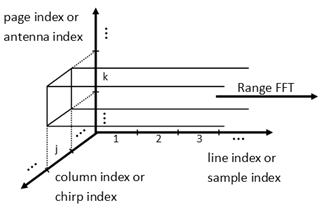Fig. 2: Range FFT input data processing

# DATA PROCESSING FLOWCHART

Because the demodulated signal is real, the range FFT can be sped-up by computing the DFTs of two real signals at once. This is done by computing the DFT of the complex signal which has on the real part the first signal and on the imaginary part the second one, and then split the resulted DFT.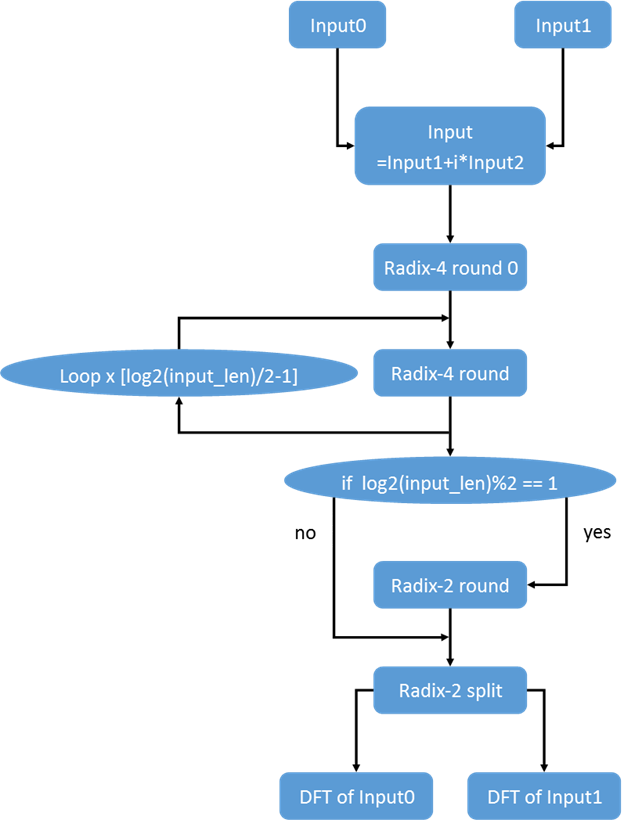Fig. 3: FFT algorithm data processing flowchart

FFT INPUT DATA

The FFT input data is made of the second chirp of the first two antennas.

% First real input is the second chirp from first antenna

input0 = input_data_rs(:, 2, 1);

% Second real input is the second chirp from second antenna

input1 = input_data_rs(:, 2, 2);

input = input0 + 1i * input1;

Below are the two real inputs. It can be observed that the two inputs are sinusoids with the same frequency, but different phase.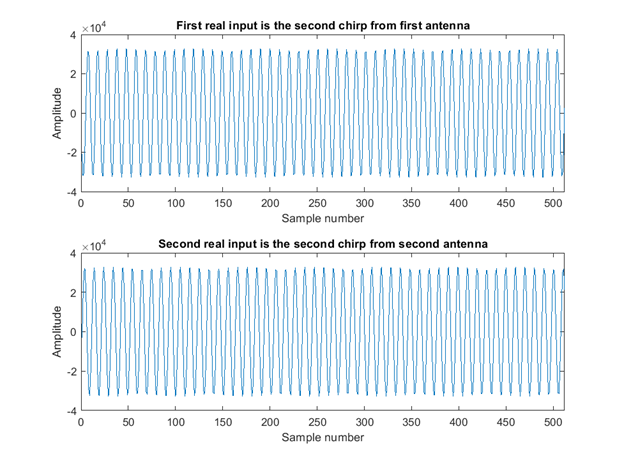Fig. 4: FFT input data

FFT PROCESSING

One radix-4 is done using the instruction RDX4, and one radix-2 round is done using the instruction RDX2. The first round of the FFT is a radix-4 round. In this round, because there is no need of twiddle factors, the instruction RDX4 lets you apply a window function on the input sequence. After we apply the remaining radix-4 rounds, if needed, we apply one radix-2 round. The last step is to find the DFTs of the real signals. This is done using the instruction RDX2.

% Deduce the number of radix-4 rounds and if there is a radix-2 round

input_length = numel(input);

log2_input_length = log2(input_length);

num_rdx4_rounds = floor(log2_input_length / 2);

num_rdx2_rounds = mod(log2_input_length, 2);

num_rounds = num_rdx4_rounds + num_rdx2_rounds;

% Twiddle factors

t = (1:input_length/8)/input_length;

M_Tw_real = round_and_saturate(cos(-2*pi*t), NBITS) * 2^(NBITS-1);

M_Tw_imag = round_and_saturate(sin(-2*pi*t), NBITS) * 2^(NBITS-1);

M_Tw = M_Tw_real + 1i * M_Tw_imag;

% Results

M_Out = zeros(num_rounds + 1, input_length);

% Windowing operation - Indicates whether or not is the window multiplication

% enabled

win_type = 'WIN_DISABLED';

% Win coefficient type - Indicates the type of window coefficients

win_coeff_type = 'MULTIPLE_COEFF'; % do not care

% Repeat - Decides whether combined fft operation is enabled or not

repeat = 'NO_COMBINED_FFT';

% Pre-scaling left shift value

shft_val = 0;

% FFT Round

fft_rnd = 0;

% Window function

M_Wc = 0;

% Operands

M_Op = input;

% Result

M_Out(1, : )= rdx4_mex(complex(M_Wc), complex(M_Op), win_type, win_coeff_type, fft_rnd, quad_ext, repeat, shft_val);

for i = 1:num_rdx4_rounds-1

% FFT Round

fft_rnd = i;

% Operands values

M_Op = M_Out(i, :);

% Result

M_Out(i+1, : )= rdx4_mex(M_Tw, M_Op, win_type, win_coeff_type, fft_rnd, quad_ext, repeat, shft_val);

end

% rdx2 round

if num_rdx2_rounds ~= 0

% FFT Round

fft_rnd = num_rounds - 1;

% Real FFT

real_fft = 'NO_OPERANDS_SPLIT';

% Repeat

repeat = 'NO_COMBINED_FFT';

% Pre-scaling left shift value

shft_val = 0;

% Operands values

M_Op = M_Out(num_rounds - 1, :);

% Result

M_Out(num_rounds, : )= rdx2_mex(M_Tw, M_Op, fft_rnd, real_fft, quad_ext, repeat, shft_val);

end

% DFT split

% FFT Round

fft_rnd = num_rounds - 1;

% Real FFT

real_fft = 'OPERANDS_SPLIT';

% Repeat

repeat = 'NO_COMBINED_FFT';

% Pre-scaling left shift value

shft_val = 0;

% Operands values

M_Op = M_Out(num_rounds, :);

% Result

M_Out(end, : )= rdx2_mex(M_Tw, M_Op, fft_rnd, real_fft, quad_ext, repeat, shft_val);

RESULTS

We verify the results using the FFT MATLAB function. In the image below the first column displays the magnitude of the two DFTs, and the second column displays their phases.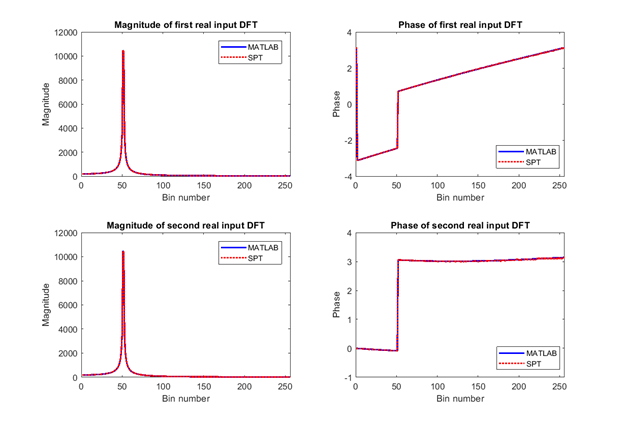Fig. 5: Magnitude and Phase DFT of the real input signals

The script used in this example is attached below.

We hope you find this information useful. Feel free to LIKE this article and comment below.

0 Replies